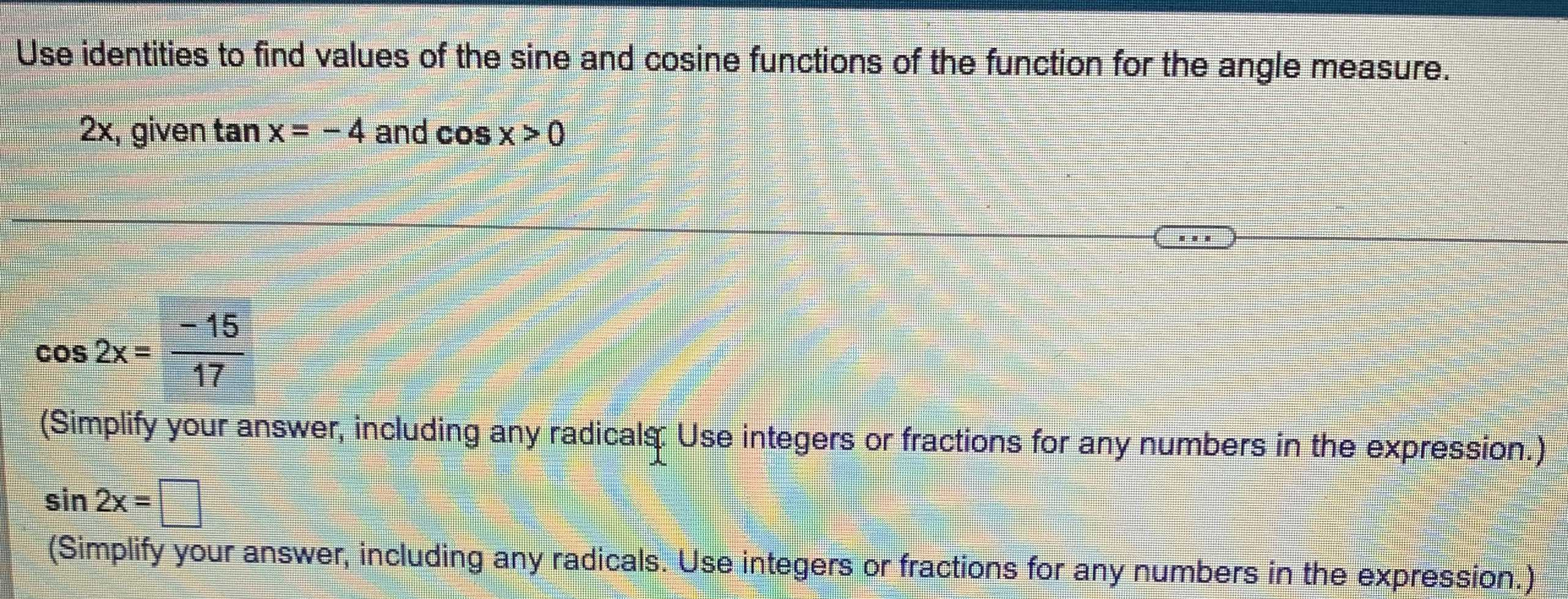### Still have math questions?

Trigonometry
QuestionUse identities to find values of the sine and cosine functions of the function for the angle measure.

$$2 x$$ , given $$\tan x = - 4$$ and $$\cos x > 0$$

$$\cos 2 x = \frac { - 15} { 17}$$

$$\sin 2 x = \square$$
$$\frac { - 8} { 27}$$